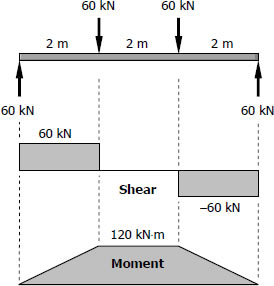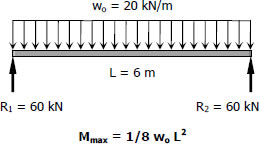# Wide-Flange

## For Girders (G - 2)$S_{required} = \dfrac{M}{f_b} = \dfrac{120(1000^2)}{120}$
$S_{required} = 1000 \times 10^3 \, \text{mm}^3$

## For Beams (B - 3)$M_{max} = \frac{1}{8}(20)(62)$
$M_{max} = 90 \, \text{kN}\cdot\text{m}$

$S_{required} = \dfrac{M_{max}}{f_b} = \dfrac{90(1000^2)}{120}$
$S_{required} = 750 \times 10^3 \, \text{mm}^3$

## Pages